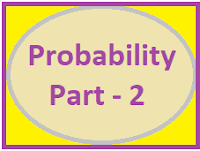### Common Errors in Secondary Mathematics

Common Errors Committed  by the  Students  in Secondary Mathematics   Errors  that students often make in doing secondary mathematics  during their practice and during the examinations  and their remedial measures are well explained here stp by step.  Some Common Errors in Mathematics

### PROBABILITY Part-2

PROBABILITY PART-2This part is the continuation of Probability part-1. This Post is specially for the students after 10th standard. For complete knowledge first students study Probability Part-1 and then part-2
 Main points to be discussed here 1 Random Experiment 2 Different types of events 3 Variable description of the events 4 Conditional Probability 5 Multiplication Theorem on Probability 6 Laws of Total Probability 7 Baye's Theorem 8 Bernoulli's Trial 9 Mean and Variance of the Distribution 10 Shortcut Method of finding Mean, Variance and Standard Deviation.

Random Experiment:-
An experiment whose outcomes cannot be predicted or determined in advance are called a random experiment.

Elementary events:-
If a random experiment performed, then each of its outcome is known as an elementary event.

Sample space:-
The set of all possible outcomes of a random experiment is called the sample space.

Event:- A subset of the sample space is called an event.

Simple Event :-
An event having only one sample point is called the simple event. eg:- HH, TT, HHH, 222 .... etc.
Compound event:-
An event having more than one sample point is called a compound event. eg:- HT, HTH,  123 ... etc.

Mutually exclusive events:-
Two or more events are said to be mutually exclusive events if their intersection is a null set eg:- A and B are said to be mutually exclusive events If A∩B = Φ

Exhaustive events:-
Two or more events are said to be exhaustive events, if their union is a sample space, For Example:-  A ∪ B ∪ C ∪ ..... = S

Conditional probability:-
Conditional probability of two events A and B is denoted by P(A|B) and is called as
P(A|B) = Probability of occurrence  of A given that B has already occurred.

Conditional Probability is calculated by using following formula.
 $P(A|B)=\frac{P(A\cap B)}{P(B)}$

Independent events:- If A and B are independent events then
 $P(A\cap B)=P(A).P(B)$ $P(A\cap B\cap C)=P(A).P(B).P(C)$

Explanation of Some symbols and Terms used in the problems
 Variable Description of the event ⇒ Set theory notation A or B (At least one of A or B) = A ∪ B A and B = A ∩ B ,                    Not A = Ā $A \: but \: not\: B = A\cap\overline{B}$ $Either\; A\: or\: B=A\cup B$ $Neither\: A\: nor\: B=\overline{A}\cap \overline{B}$ $All \: three\: of\: A, B \: and \: C = A\cap B\cap C$ $At\: least\: one\: of\: A, B, or\: C=A\cup B\cup C$ $Exactly \: \: one\: of\: A\: and\: B = (A\cap \overline{B})\cup (\overline{A}\cap B)$ $D' Morgan's Law:-\: A'\cap B'=(A\cup B)'$$OR\: \: \: A'\cup B'=(A\cap B)'$ $P(A\cup B)=P(A)+P(B)-P(A\cap B)$$P(A\cup B\cup C)=P(A)+P(B)+P(C)-P(A\cap B)$$-P(B\cap C)-P(C\cap A)+P(A\cap B\cap C)$
************************************
 Multiplication Theorem On Probability If A and B are two events associated with a random experiment, then $P(A\cap B)=P(A)P(B/A)\: where P(A)\neq 0$ $P(A\cap B)=P(B)P(A/B)\: where P(B)\neq 0$ Extension of Multiplication Theorem $If A_{1},A_{2},A_{3},.....,A_{n}\: are\: n \: events\: associated\: with\: the\: random\: experiment,\: then$ $P(A_{1}\cap A_{2}\cap A_{3}.....\cap A_{n})=P(A_{1})P(A_{2}/A_{1})P(A_{3}/A_{1}\cap A_{2})P(A_{4}/A_{1}\cap A_{2}\cap A_{3}).....$ $........P(A_{n}/A_{1}\cap A_{2}\cap .....\cap A_{n-1})$
The laws of total probability:-
$Let\: S\: \: be\: the\: sample\: space \: and \: let\: E_{1},E_{2},E_{3},....,E_{n} \: be \: n \: mutually\: exclusive$$and\: exhaustive\: events.$$If \: A \: is \: any\: event,\: then$$P(A)=P(E_{1})P(A/E_{1})+P(E_{2})P(A/E_{2})+.....+P(E_{n})P(A/E_{n})$
 BAYE'S THEOREM $Let \: S\: be\: the \: sample\: space \: and\: let\: E_{1},E_{2},E_{3},.....,E_{n}\: be$ $mutually \: exclusive\: and\: exhaustive\: events\: associated\: with\: a\: random\: experiment.$ $If\: A \: is\: any \: event\: which\: occurs\: with\: E_{1} \: or\: E_{2}\: or\: E_{3}\: or...or\: E_{n}$ $P(E_{i}|A)=\frac{P(E_{i})P(A|E_{i})}{\sum P(E_{i})P(A|E_{i})}\: \: i=1,2,3....,n$If n = 2 then Baye's Theorem written as: $P(E_{1}|A) =\frac{P(E_{1})P(A|E_{1})}{P(E_{1})P(A|E_{1}) +P(E_{2}) P(A|E_{2})}$$P(E_{2}|A)=\frac{P(E_{2})P(A|E_{2})}{P(E_{1})P(A|E_{1})+ P(E_{2}) P(A| E_{2})}$If n = 3 then Baye's Theorem written as:$P(E_{1}|A)=\frac{P(E_{1})P(A|E_{1})}{P(E_{1})P(A|E_{1})+P(E_{2})P(A|E_{2}) +P(E_{3})P(A|E_{3})}$$P(E_{2}|A)=\frac{P(E_{2})P(A|E_{2})}{P(E_{1})P(A|E_{1})+P(E_{2})P(A|E_{2}) +P(E_{3})P(A|E_{3})}$ $P(E_{3}|A)=\frac{P(E_{3})P(A|E_{3})}{P(E_{1})P(A|E_{1})+P(E_{2})P(A|E_{2}) +P(E_{3})P(A|E_{3})}$
MEAN AND VARIANCE OF THE DISTRIBUTION
 $Mean(\overline{X})=\sum p_{i}x_{i}\: \: \: where\: \: i=1\: to\: n$$Variance(X) = \sum p_{i}x_{i}^{2}\: -\: \left ( \sum p_{i}x_{i} \right )^{2}\: \: \: where\: \: i=1\: to\: n$

BERNOULLI TRIAL
A trial of a random experiment are called Bernoulli trial, if they satisfy the following conditions:-

a) They are finite in number.

b) They are independent of each other.

c) Each trial has exactly two outcome: success or failure.

d) The probability of success or failure remain same in each trial.

BINOMIAL DISTRIBUTION
A random variable X which takes values 0,1,2,........,n is said to follow binomial distribution
if its probability distribution function is given by
 $P(X=r)=\:^{n}C_{r}\: p^{r}q^{n-r}, where r=1,2,3....n$
Where p and q are the probability of the two events such that   p + q = 1

n is the number of trials and r is the random variable .

The two constants n and p are called parameters of the distribution
$P(X=0)+P(X=1)+.....+P(X=n)=^{n}C_{0}p^{0}q^{n-0}+^{n}C_{1}p^{1}q^{n-1}.....^{n}C_{n}p^{n}q^{0}$
$\: \: \: \: \: \: =(p+q)^{n}=1^{n}=1$
 Bernoulli’s Trial Probability of r successes in ‘n’ Bernoulli trial is given by $P(r\: successes)=^{n}C_{r}p^{r}q^{n-r}$ $P(r\:successes)=\frac{n!}{r!(n-r)!}.p^{r}q^{n-r}$ Where  n = number of trials r = number of successful trials = 0, 1, 2, 3, ………., n p = probability of success in a trial q = probability of failure in a trial and  p + q = 1

The probability distribution of number of successes for a random variable X can be written as:

This probability distribution is called Binomial distribution with parameters n and p.

 Shortcut Method of finding Mean, Variance and Standard Deviation The binomial distribution with n Bernoulli trials and success p is also denoted by B(n, p)Where ‘n’ number of Bernoulli trial  p denotes the probability of success  q denotes the probability of failure then, Mean =  np Variance = npq Standard Deviation = Square root of  npq

🐉🙏🐉